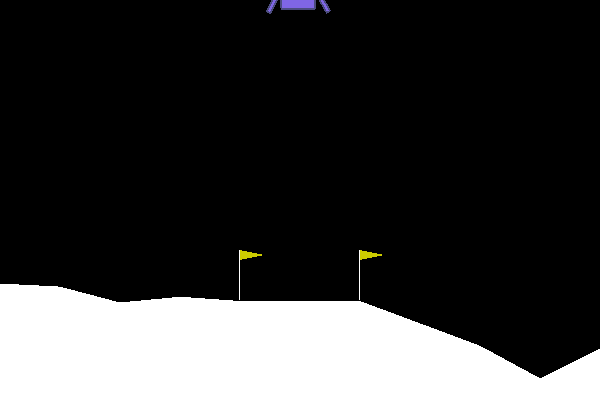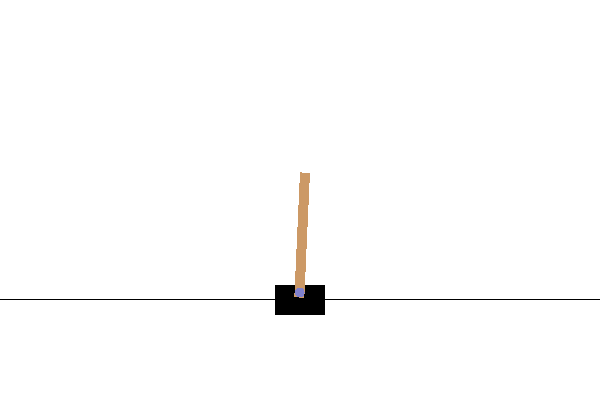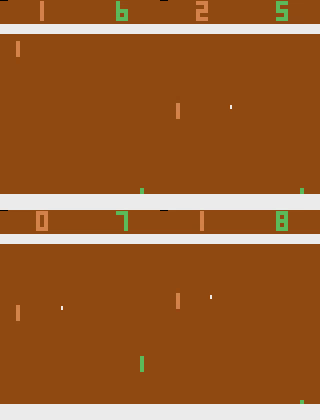# Examples¶

## Try it online with Colab Notebooks!¶

All the following examples can be executed online using Google colabnotebooks:

In the following example, we will train, save and load an A2C model on the Lunar Lander environment.Lunar Lander Environment

Note

LunarLander requires the python package box2d. You can install it using apt install swig and then pip install box2d box2d-kengz

import gym

from stable_baselines.common.policies import MlpPolicy
from stable_baselines.common.vec_env import DummyVecEnv
from stable_baselines import A2C

# Create and wrap the environment
env = gym.make('LunarLander-v2')
env = DummyVecEnv([lambda: env])

model = A2C(MlpPolicy, env, ent_coef=0.1, verbose=1)
# Train the agent
model.learn(total_timesteps=100000)
# Save the agent
model.save("a2c_lunar")

# Enjoy trained agent
obs = env.reset()
for i in range(1000):
action, _states = model.predict(obs)
obs, rewards, dones, info = env.step(action)
env.render()


## Multiprocessing: Unleashing the Power of Vectorized Environments¶CartPole Environment

import gym
import numpy as np

from stable_baselines.common.policies import MlpPolicy
from stable_baselines.common.vec_env import SubprocVecEnv
from stable_baselines.common import set_global_seeds
from stable_baselines import ACKTR

def make_env(env_id, rank, seed=0):
"""
Utility function for multiprocessed env.

:param env_id: (str) the environment ID
:param num_env: (int) the number of environments you wish to have in subprocesses
:param seed: (int) the inital seed for RNG
:param rank: (int) index of the subprocess
"""
def _init():
env = gym.make(env_id)
env.seed(seed + rank)
return env
set_global_seeds(seed)
return _init

env_id = "CartPole-v1"
num_cpu = 4  # Number of processes to use
# Create the vectorized environment
env = SubprocVecEnv([make_env(env_id, i) for i in range(num_cpu)])

model = ACKTR(MlpPolicy, env, verbose=1)
model.learn(total_timesteps=25000)

obs = env.reset()
for _ in range(1000):
action, _states = model.predict(obs)
obs, rewards, dones, info = env.step(action)
env.render()


## Using Callback: Monitoring Training¶

You can define a custom callback function that will be called inside the agent. This could be useful when you want to monitor training, for instance display live learning curves in Tensorboard (or in Visdom) or save the best agent.Learning curve of DDPG on LunarLanderContinuous environment

import os

import gym
import numpy as np
import matplotlib.pyplot as plt

from stable_baselines.ddpg.policies import MlpPolicy
from stable_baselines.common.vec_env.dummy_vec_env import DummyVecEnv
from stable_baselines.bench import Monitor
from stable_baselines import DDPG

best_mean_reward, n_steps = -np.inf, 0

def callback(_locals, _globals):
"""
Callback called at each step (for DQN an others) or after n steps (see ACER or PPO2)
:param _locals: (dict)
:param _globals: (dict)
"""
global n_steps, best_mean_reward
# Print stats every 1000 calls
if (n_steps + 1) % 1000 == 0:
# Evaluate policy performance
if len(x) > 0:
mean_reward = np.mean(y[-100:])
print(x[-1], 'timesteps')
print("Best mean reward: {:.2f} - Last mean reward per episode: {:.2f}".format(best_mean_reward, mean_reward))

# New best model, you could save the agent here
if mean_reward > best_mean_reward:
best_mean_reward = mean_reward
# Example for saving best model
print("Saving new best model")
_locals['self'].save(log_dir + 'best_model.pkl')
n_steps += 1
return False

# Create log dir
log_dir = "/tmp/gym/"
os.makedirs(log_dir, exist_ok=True)

# Create and wrap the environment
env = gym.make('LunarLanderContinuous-v2')
env = Monitor(env, log_dir, allow_early_resets=True)
env = DummyVecEnv([lambda: env])

# Add some param noise for exploration
model = DDPG(MlpPolicy, env, param_noise=param_noise, memory_limit=int(1e6), verbose=0)
# Train the agent
model.learn(total_timesteps=200000, callback=callback)


## Atari Games¶Trained A2C agent on BreakoutPong Environment

Training a RL agent on Atari games is straightforward thanks to make_atari_env helper function. It will do all the preprocessing and multiprocessing for you.from stable_baselines.common.cmd_util import make_atari_env
from stable_baselines.common.policies import CnnPolicy
from stable_baselines.common.vec_env import VecFrameStack
from stable_baselines import ACER

# There already exists an environment generator
# that will make and wrap atari environments correctly.
# Here we are also multiprocessing training (num_env=4 => 4 processes)
env = make_atari_env('PongNoFrameskip-v4', num_env=4, seed=0)
# Frame-stacking with 4 frames
env = VecFrameStack(env, n_stack=4)

model = ACER(CnnPolicy, env, verbose=1)
model.learn(total_timesteps=25000)

obs = env.reset()
while True:
action, _states = model.predict(obs)
obs, rewards, dones, info = env.step(action)
env.render()


## Mujoco: Normalizing input features¶

Normalizing input features may be essential to successful training of an RL agent (by default, images are scaled but not other types of input), for instance when training on Mujoco. For that, a wrapper exists and will compute a running average and standard deviation of input features (it can do the same for rewards).

Note

We cannot provide a notebook for this example because Mujoco is a proprietary engine and requires a license.

import gym

from stable_baselines.common.policies import MlpPolicy
from stable_baselines.common.vec_env import DummyVecEnv, VecNormalize
from stable_baselines import PPO2

env = DummyVecEnv([lambda: gym.make("Reacher-v2")])
# Automatically normalize the input features
env = VecNormalize(env, norm_obs=True, norm_reward=False,
clip_obs=10.)

model = PPO2(MlpPolicy, env)
model.learn(total_timesteps=2000)

# Don't forget to save the running average when saving the agent
log_dir = "/tmp/"
model.save(log_dir + "ppo_reacher")
env.save_running_average(log_dir)


## Custom Policy Network¶

Stable baselines provides default policy networks for images (CNNPolicies) and other type of inputs (MlpPolicies). However, you can also easily define a custom architecture for the policy network (see custom policy section):

import gym

from stable_baselines.common.policies import FeedForwardPolicy
from stable_baselines.common.vec_env import DummyVecEnv
from stable_baselines import A2C

# Custom MLP policy of three layers of size 128 each
class CustomPolicy(FeedForwardPolicy):
def __init__(self, *args, **kwargs):
super(CustomPolicy, self).__init__(*args, **kwargs,
layers=[128, 128, 128],
feature_extraction="mlp")

# Create and wrap the environment
env = gym.make('LunarLander-v2')
env = DummyVecEnv([lambda: env])

model = A2C(CustomPolicy, env, verbose=1)
# Train the agent
model.learn(total_timesteps=100000)


## Continual Learning¶

You can also move from learning on one environment to another for continual learning (PPO2 on DemonAttack-v0, then transferred on SpaceInvaders-v0):

from stable_baselines.common.cmd_util import make_atari_env
from stable_baselines.common.policies import CnnPolicy
from stable_baselines import PPO2

# There already exists an environment generator
# that will make and wrap atari environments correctly
env = make_atari_env('DemonAttackNoFrameskip-v4', num_env=8, seed=0)

model = PPO2(CnnPolicy, env, verbose=1)
model.learn(total_timesteps=10000)

obs = env.reset()
for i in range(1000):
action, _states = model.predict(obs)
obs, rewards, dones, info = env.step(action)
env.render()

# The number of environments must be identical when changing environments

# change env
model.set_env(env)
model.learn(total_timesteps=10000)

obs = env.reset()
while True:
action, _states = model.predict(obs)
obs, rewards, dones, info = env.step(action)
env.render()


## Bonus: Make a GIF of a Trained Agent¶

Note

For Atari games, you need to use a screen recorder such as Kazam. And then convert the video using ffmpeg

import imageio
import numpy as np

from stable_baselines.common.policies import MlpPolicy
from stable_baselines import A2C

model = A2C(MlpPolicy, "LunarLander-v2").learn(100000)

images = []
obs = model.env.reset()
img = model.env.render(mode='rgb_array')
for i in range(350):
images.append(img)
action, _ = model.predict(obs)
obs, _, _ ,_ = model.env.step(action)
img = model.env.render(mode='rgb_array')

imageio.mimsave('lander_a2c.gif', [np.array(img) for i, img in enumerate(images) if i%2 == 0], fps=29)